# HC Verma Solutions Class 11 Chapter 16 Sound Waves

HC Verma Class 11 Solutions Chapter 16 Sound waves will help students in solving different questions related to the velocity of sound in air and solid and more. They will further learn how to solve questions related to finding time interval when velocity and distance are given and other important chapter questions that are frequent in competitive exams such as IIT JEE. In order to help students understand the concepts clearly and develop better problem-solving skills, we are providing free HC Verma Solutions pdf here. Students will get to learn the right method of solving problems such as:

• Finding the maximum and the minimum wavelength.
• Finding the ratio between velocity amplitude, wave speed and bulk modulus of elasticity, etc.
• Finding the phase difference when the distance travelled by particles are given.
• Students will also deal with questions on finding the bulk modulus when pressure amplitude and displacement amplitude is given.
• Solve questions on destructive interference when the path difference and wavelength of sound waves are given.

These questions will help you understand this chapter in a better way. The solutions for exercises given in the HC Verma book part 1 that we are offering is crafted in such a manner to help students learn effectively and be well prepared for the exam.

Students will learn about different topics such as;

1. The Nature and Propagation of Sound Waves
2. Displacement Wave and Pressure Wave
3. Speed of a Sound Wave in a Material Medium
4. Speed of Sound in a Gas: Newton’s Formula and Laplace’s Correction
5. Effect of Pressure, Temperature and Humidity on the Speed of Sound in Air
6. Intensity of Sound Waves
7. Appearance of Sound to Human Ear
8. Interference of Sound Waves
9. Standing Longitudinal Waves and Vibrations of Air Columns
10. Determination of Speed of Sound in Air
11. Beats
12. Diffraction
13. Doppler Effect
14. Sonic Booms
15. Musical Scale
16. Acoustics of Buildings

To access all the chapters of Vol 1 and Vol 2 Click Here.

## Class 11 Important Questions In Chapter 16

1. During an experiment, we take two tuning forks that vibrate with the same amplitude. However, their frequency is not the same. The first fork has a frequency that is double of the second. According to you which fork will produce more sound in air?

2. Take a vertical rod and hit it at one end. State what kind of wave is formed if (a) the rod is hit vertically (b) horizontally?

3. Which factor will remain unchanged if the sound wave is refracted from air to water?

(a) Frequency (b) Wave Velocity (c) Wave number (d) Wavelength

4. What does the speed of sound in a medium depend upon?

(a) the elastic property

(b) the inertia property

(c) both elastic and inertia property

(d) neither the elastic property nor the inertia property.

5. The Doppler effect usually causes a change in frequency but it does not depend on

(a) the frequency of the source (b) the speed of the source (c) the speed of the observer (d) separation between the source and the observer.

## HC Verma Solutions Vol 1 Sound Waves Chapter 16

Question 1: A steel tube of length 1.00 m is struck at one end. A person with his ear close to the other end hears the sound of the blow twice, one travelling through the body of the tube and the other through the air in the tube. Find the time gap between the two hearings. Use the table in the text for speeds of sounds in various substances.

Solution:

Velocity of sound in air = v = 330 m/s

Velocity of sound through the steel tube = vs = 5200 m/s

Length of the steel tube= S = 1 m

Required time gap = t = t1 – t2

Where t1 = time taken by the sound in air = 1/330 and t2 = time taken by the sound in steel tube = 1/5200

=> t = 1/330 – 1/5200 = 2.75 ms

Question 2: At a prayer meeting, the disciples sing JAI-RAM JAI-RAM. The sound amplified by a loudspeaker comes back after reflection from a building at a distance of 80 m from the meeting. What maximum time interval can be kept between one JAI.R4M and the next JAI-RAM so that the echo does not disturb a listener sitting in the meeting. Speed of sound in air is 320 m s-1.

Solution:

S = (80)(2) = 160 m

v= 320 m/s

So, maximum time interval is: t = S/v = 160/320 = 0.5 sec

Question 3: A man stands before a large wall at a distance of 50.0 m and claps his hands at regular intervals. Initially, the interval is large. He gradually reduces the interval and fixes it at a value when the echo of a clap merges with the next clap. If he has to clap 10 times during every 3 seconds, find the velocity of sound in air.

Solution:

S = 50 m

Man has to clap 10 times in 3 sec

So, the time interval between two claps = 3/10

Time taken by the sound to reach the wall = t = 3/20 sec

Velocity = v = S/t

= 50/(3/20)

= 333 m/sec

Question 4: A person can hear sound waves in the frequency range 20 Hz to 20 kHz. Find the minimum and the maximum wavelengths of sound that is audible to the person. The speed of sound is 360 m s-1.

Solution:

Speed of sound =v= 360 m/sec

Frequency for minimum wavelength, f = 20 kHz

We know, v = fλ

or λ = 18 x 10-3 mm

Again, Frequency for max. wavelength, f = 20 Hz

λ = 360/20 = 18 m

Question 5: Find the minimum and maximum wavelengths of sound in water that is in the audible range (20—20000 Hz) for an average human ear. Speed of sound in water = 1450 m s-1.

Solution:

Speed of sound =v= 1450 m/sec

For minimum wavelength, frequency should be max.

f = 20 kHz

We know, v = fλ

or λ = 1450/[20×103] = 7.25 cm

For minimum wavelength, frequency should be min.

λ = 20 Hz

v = fλ

λ = 1450/20 = 72.5 m

Question 6: Sound waves from a loudspeaker spread nearly uniformly in all directions if the wavelength of the sound is much larger than the diameter of the loudspeaker.

(a) Calculate the frequency for which the wavelength of sound in air is ten times the diameter of the speaker if the diameter is 20 cm.

(b) Sound is essentially transmitted in the forward direction if the wavelength is much shorter than the diameter of the speaker. Calculate the frequency at which the wavelength of the sound is one tenth of the diameter of the speaker described above. Take the speed of sound to be 340 m s-1.

Solution:

Wavelength of the sound is 10 times the diameter of the loudspeaker.

λ = 20 x 10 = 200 cm or 2 m

(a) v = fλ

f = v/λ = 340/2 = 170 Hz

λ = 2 cm = 2 x 10-2 m

f =v/λ = 340/(2×10-2) = 17000 Hz = 17 k Hz

Question 7: Ultrasonic waves of frequency 4.5 MHz are used to detect tumour in soft tissues. The speed of sound in tissue is 1.5 km s-1 and that in air is 340 m s-1. Find the wavelength of this ultrasonic wave in air and in tissue.

Solution: Frequency of ultrasonic wave = f= 4.5 MHz or 4.5 x 106 Hz

Speed of sound in tissue = 1.5 km/s

Velocity of air v = 340 m/sec

v= fλ

λ = 340/[4.5×106]

= 7.6 x 10-5 m

(b) Velocity of sound in tissue:

vt = 1500 m/s

λ = vt f

λ = 1500/[4.5×106] m

= 3.3 x 10-4 m

Question 8: The equation of a travelling sound wave is y = 6.0 sin(600t – 1.8x) where y is measured in 10-5 m, t in second and x in metre. (a) Find the ratio of the displacement amplitude of the particles to the wavelength of the wave. (b) Find the ratio of the velocity amplitude of the particles to the wave speed.

Solution:

Given: ry = 6.0 x 10-5 m

(a) 2π/λ = 1.8

=> λ = 2π/1.8

So, ry/λ = [6.0 x 10-5 x 1.8]/2π

= 1.7 x 10-5 m

(b) Let vy be velocity amplitude.

v = dy/dt = 3600 cos(600t -1.8)x10-5 m/s

Here vy = 3600 x 10-5 m/s

and λ = 2π/1.8 and T = 2π/600

=> wave speed = v = λ/T = 600/1.8 = 1000/3 m/s

So, the ratio = vy/v = [3600x3x10-5]/1000

Question 9: A sound wave of frequency 100 Hz is travelling in air. The speed of sound in air is 350 m s-1. (a) By how much is the phase changed at a given point in 2.5 ms? (b) What is the phase difference at a given instant between two points separated by a distance of 10.0 cm along the direction of propagation?

Solution:

(a) v = fλ

λ = v/f = 350/100 = 3.5 m

In 2.5 ms, the distance travelled by the particle,

Δx = (350 x 2.5 x 10-3)m

Now, phase difference = φ = (2π/λ) Δx

= [2πx350x2.5×10-3]/[3.5]

=> φ = π/2

(b) Distance between the two points:

Δx = 10 cm = 0.1 m

φ = (2π/λ) Δx

On substituting the values,

φ = (2π(0.1)/3.5 = 2π/35

Phase difference between the two points.

Question 10: Two point sources of sound are kept at a separation of 10 cm. They vibrate in phase to produce waves of wavelength 5.0 cm. What would be the phase difference between the two waves arriving at a point 20 cm from one source (a) on the line joining the sources and (b) on the perpendicular bisector of the line joining the sources?

Solution:

(a) Δx = 10 cm and λ = 5 cm

=> φ = (2π/λ) Δx = (2π/5)10 = 4π

Phase difference between the two waves is zero.

(b) Zero: the particles are in the same phase since they have the same path.

Question 11: Calculate the speed of sound in oxygen from the following data. The mass of 22.4 litre of oxygen at STP (T = 273 K and p = 1.0 × 105 N m-2) is 32 g, the molar heat capacity of oxygen at constant volume is Cv = 2.5 R and that at constant pressure is Cp = 3.5 R

Solution:

p = 1 x 105 N/m2, T = 273 K, M = 32 g and g = 32×10-3 kg

v = 22.4 l = 22.4 x10-3 m3

Therefore, C/Cv = r = 3.5R/2.5R = 1.4

V = √(rp/f) = [1.4×1.0x10-5]/[32/22.4] = 310 m/s

Question 12: The speed of sound as measured by a student in the laboratory on a winter day is 340 m s-1 when the room temperature is 17°C. What speed will be measured by another student repeating the experiment on a day when the room temperature is 32°C?

Solution:

velocity of sound = v1 = 340 m/s

T1 = 17o C = 17+273 = 290 K

Let v2 velocity of sound at temp T2

T2 = 32o C = 32 + 273 = 305 K

Relation between velocity and temperature:

v ∝ √T

Now, v1/v2 = √T1/√T2

=> v2 = 340 x √(305/290) = 349 m/s

The final velocity of sound is 349 m/s.

Question 13: At what temperature will the speed of sound be double of its value at 0°C?

Solution:

T1 = 273 , v2 = 2v1, v1 = v and T2 = ?

We know that, v ∝ √T

=> T2/T1 = v22/v12

=> T2 = 273 x 22 = 4 x 273 K

So, temp will be (4 x 273) – 273 = 819o C

Question 14: The absolute temperature of air in a region linearly increases from T1 to T2 in a space of width d. Find the time taken by a sound wave to go through the region in terms of T1, T2, d and the speed u of sound at 273 K. Evaluate this time for T1 = 280 K, T2 = 310 K, d = 33m and u = 330 m s-1.

Solution:

Temperature variation:

T = T1 + [(T2 – T1)x]/d

v ∝ √T

vT/v = √(T/273)

And, dt = dx/vT = du/v (√(273/T))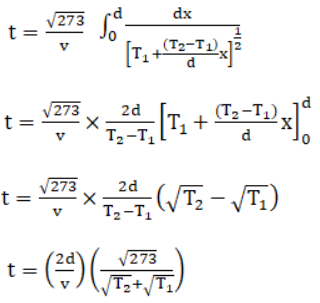We are given, initial temp = T1 = 280k

Final temp = T2 = 310 k

Space width = d = 33 m

and v = 330 m/s

=> T = [2x33x√273]/[330(√280+√310)]

= 96 m/s

Question 15: Find the change in the volume of 1.0 liter kerosene when it is subjected to an extra pressure of 2.0 × 105 N m-2 from the following data. Density of kerosene = 800 kg m-3 and speed of sound in kerosene 1330 m s-1.

Solution:

The velocity in terms of the bulk modulus and density :

v = √(k/p)

where, k = v2 ρ

=> k = (1330) 2 x 800 N/m2

K = (F/A)/(Δv/Δv)

Therefore, ΔV = [pressure x v]/k

Δv = [2×105x1x10-3]/[1330x1330x800]

= 0.14 cm3

The change in the volume of kerosene 0.14 cm3.

Question 16: Calculate the bulk modulus of air from the following data about a sound wave of wavelength 35 cm travelling in air. The pressure at a point varies between (1.0 × 105 ± 14) pa and the particles of the air vibrate in simple harmonic motion of amplitude 5.5 × 10-6 m.

Solution:

Wavelength of sound wave = λ = 35 cm = 35 x 10-2 m

Pressure amplitude = po = (1 x 105 ± 14) pa

Displacement amplitude of the air particles =So = 5.5 x 10-6 m

Now,

Bulk modulus of air = B = poλ/2πSo = Δp/(Δ v/v)

= [14x35x10-2]/[2π(5.5 x 10-6)]

= 1.4 x 105 N/m2

Question 17: A source of sound operates at 20 kHz, 20 W emitting sound uniformly in all directions. The speed of sound in air is 340 m s-1 and the density of air is 1.2 kg m-3.

(a) What is the intensity at a distance of 60 m from the source?

(b) What will be the pressure amplitude at this point?

(c) What will be the displacement amplitude at this point?

Solution:

(a) Distance of the source = r = 6.0 m

Intensity = I = P/A

here P = 20 W and A = area = 4πr2

=> I = 20/[4πr2]

Given r = 6 m

=> I = 44 mw/m2

(b) I = po/2ρv

=> po = √(2Iρv)

=> po = √(2x12x340x44x10-3)

=> po = 6 Pa

(c) As, I = 2π2So2 v2 ρv

Where So is the displacement amplitude

s_o = √(I/2π2v2 ρv)

on substituting the values, we get

So = 1.2 x 10-6 m

Question 18: The intensity of sound from a point source is 1.0 × 10-8 W m-2 at a distance of 5.0 m from the source. What will be the intensity at a distance of 25 m from the source?

Solution:

Here l1 = 1 x 10-8 W m-2

r1 = 5 m and r2 = 25 m

l2 =?

We know, l ∝ 1/r2

=> l1r12 = l2r22

=> l2 = (l1r12)/r22

= [1×10-8x25]/

= 4 x 10-10 W m-2

Question 19: The sound level at a point 5.0 m away from a point source is 40 dB. What will be the level at a point 50 m away from the source?

Solution:

Sound level = β = 10 log10 (l/lo)

As per given statement,

βA = 10 log10 (lA/lo)

=> lA/lo = 10(β_A/10) …(1)

Again,

βB = 10 log10 (lB/lo)

=> lB/lo = 10(β_B/10) …(2)

From (1) and (2)

lA/lB = 10(βA – βB)/10) …(3)

Also,

lA/lB = rB2/ rA2 = (50/5)2 = 100 ….(4)

From (3) and (4),

102 = 10(βA – βB)/10)

=>2 = (βA – βB)/10

=> βA – βB = 20

=> βB = 40-20 = 20 dB

Therefore, sound level of a point 50 m away from the point source is 20 dB.

Question 20: If the intensity of sound is doubled, by how many decibels does the sound level increase?

Solution:

Sound level β1:

β1 = 10 log10 (l/lo)

Where, lo is constant reference intensity

When the intensity doubles, the sound level:

β2 = 10 log10 (2l/lo)

=> β2 – β1 = 10 log(2l/l) = 10 x 0.3010 = 3 dB

Thus, sound level is increased by 3 dB.

Question 21: Sound with intensity larger than 120 dB appears painful to a person. A small speaker delivers 2.0 W of audio output. How close can the person get to the speaker without hurting his ears?

Solution:

If sound level = 120 dB then l = intensity = 1 W/m2

Audio output = 2W (given)

Let x be the closest distance.

So, intensity = (2/4πx2) = 1

=> x2 = 2/2π

=> x = 0.4 m or 40 cm

Question 22: If the sound level in a room is increased from 50 dB to 60 dB, by what factor is the pressure amplitude increased?

Solution:

Constant reference intensity = Io = 10-12 W/m2

The initial intensity is:

β1 = 10 log10 (l1/lo)

Where, lo is constant reference intensity

50 = 10 log10 (l1/10-12)

=>I1 = 10-7 W/m2

Similarly, β2 = 10 log10 (l2/lo)

=>I2 = 10-6 W/m2

Again,

I2/ I1 = (p2/p1)2 = 10-6/10-7 = 10

Therefore, (p2/p1) = √10.

The pressure amplitude is increased by factor √10.

Question 23: The noise level in a classroom in absence of the teacher is 50 dB when 50 students are present. Assuming that on the average each student outputs same sound energy per second, what will be the noise level if the number of students is increased to 100?

Solution: Let I be the intensity of each student.

As per question,

β A = 10 log10 (50I/lo) and β B = 10 log10 (100 I/lo)

Where, lo is constant reference intensity

Now,

β B – β A = 10 log10 (100 I/50 I)

= 10 log10(2) = 3

So, β A = 50 +3 = 53 dB

Question 24: In Quincke’s experiment the sound detected is changed from a maximum to a minimum when the sliding tube is moved through a distance of 2.50 cm. Find the frequency of sound if the speed of sound in air is 340 m s-1.

Solution:

Distance between maximum and minimum:

λ/4 = 2.50 cm

=> λ = 2.50 x 4 = 10 cm = 10-1 m

As we know, v = fλ

or f = v/λ

=> f = 340/10-1 = 3400 = 3.4 kHz

Therefore, the frequency of the sound is 3.4 kHz.

Question 25: In Quincke’s experiment, the sound intensity has a minimum value I at a particular position. As the sliding tube is pulled out by a distance of 16.5 mm, the intensity increases to a maximum of 9I. Take the speed of sound in air to be 330 m/s. (a) Find the frequency of the sound source. (b) Find the ratio of the amplitudes of the two waves arriving at the detector assuming that it does not change much between the positions of minimum intensity and maximum intensity.

Solution:

(a) λ/4 = 16.5 mm

=> λ = 16.5 x 4 = 66 mm = 66 x 10-3 m

We know, v = fλ

or f = v/λ = 340/[66×10-3] = 5 kHz

(b) Ratio of maximum intensity to minimum intensity: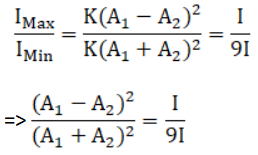(A1 + A2)/(A1 – A2) = 1/9

=> A1/A2 = 2/1

Ratio of the amplitudes is 2:1.

Question 26: Two audio speakers are kept some distance apart and are driven by the same amplifier system. A person is sitting at a place 6.0 m from one of the speakers and 64 in from the other. If the sound signal is continuously varied from 500 Hz to 5000 Hz, what are the frequencies for which there is a destructive interference at the place of the listener? Speed of sound in air = 320 m/s.

Solution:

The path difference of the two sound waves is:

ΔL = 6.4 – 6.0 = 0.4 m

The wavelength of either wave = λ = v/f = (320/f) m/s

For destructive interference,

ΔL = (2n+1)λ/2 ; n = integer

or 0.4 = (2n+1)/2 x (320/f)

[using f = 2×0.4]

=> f = (2n+1)400 Hz

On different values of n, the frequencies within the specified range that caused destructive interference are 1200 Hz, 2000 Hz, 2800 Hz, 3600 Hz and 4400 Hz.

Question 27: A source of sound S and a detector D are placed at some distance from one another. A big cardboard is placed near the detector and perpendicular to the line SD as shown in figure (below). It is gradually moved away and it is found that the intensity changes from a maximum to a minimum as the board is moved through a distance of 20 cm. Find the frequency of the sound emitted. Velocity of sound in air is 336 m/s.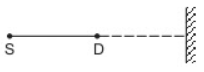Solution:

Distance between maximum and minimum intensity:

λ/4 = 20 cm

=> λ = 80 cm = 80 x 10-2 m

Let f Frequency of sound,

We know, v = fλ

Therefore, f = v/λ

= 336/[80×10-2]

= 420 Hz

Question 28: A source S and a detector D are placed at a distance d apart. A big cardboard is placed at a distance √2 d from the source and the detector as shown in figure (below). The source emits a wave of wavelength = d/2 which is received by the detector after reflection from the cardboard. It is found to be in phase with the direct wave received from the source. By what minimum distance should the cardboard be shifted away so that the reflected wave becomes out of phase with the direct wave?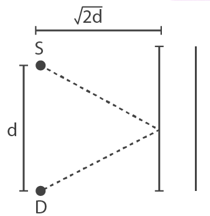Solution:

Wavelength of the source: λ = d/2

Initial path difference is 2√[(d/2)2 +2d2 – d]

If it is shifted a distance x then path difference will be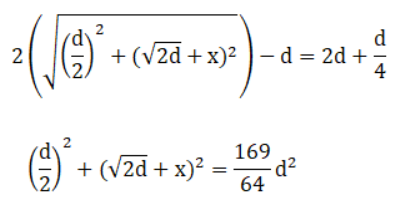[√(2d)+x]2 = [(169-16)/64 ] d2 = (153/64) d2

=> √(2d)+x = 1.54 d

or x = 1.54 d – 1.414 d = 0.13 d

Question 29: Two stereo speakers are separated by a distance of 2.40 m. A person stands at a distance of 3.20 m directly in front of one of the speakers as shown in figure (below). Find the frequencies in the audible range (20-2000 Hz) for which the listener will hear a minimum sound intensity. Speed of sound in air = 320 m/s.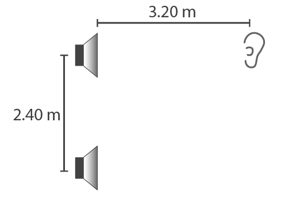Solution:

Distance between the two speakers =d = 2.40 m

Speed of sound in air=v = 320 m/sec

Find Frequency of the two stereo speakers.

Path difference between the sound waves reaching the listener:

Δ x = √[(3.2)2 + (2.4)2] = 3.2

Wavelength of either sound wave = 320/f

Now, destructive interference will occur.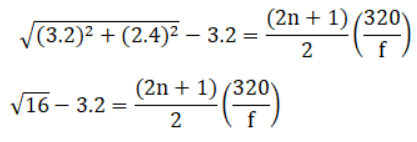1.6f = (2n + 1)320

=> f = 200(2n+1)

Where n = 1,2,3,…,49

Question 30: Two sources of sound, S1 and S2, emitting waves of equal wavelength 20.0 cm, are placed with a separation of 20.0 cm between them. A detector can be moved on a line parallel to S1S2 and at a distance of 20.0 cm from it. Initially, the detector is equidistant from the two sources. Assuming that the waves emitted by the sources are in phase, find the minimum distance through which the detector should be shifted to detect a minimum of sound.

Solution: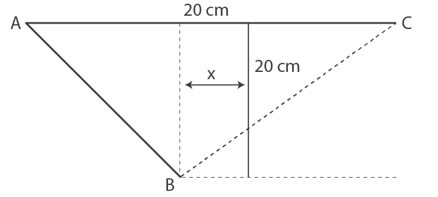Wavelength of sound wave = λ = 20 cm

Distance of detector from source BD = 20 cm

Separation between the two sources AC = 20 cm

Now, Path difference = AB-BC

= √[(202+(10+x)2] – √[(202+(10-x)2]

To hear the minimum, this path difference:

[(2n+1)λ]/2 = λ/2 = 10 cm

=> √[(202+(10+x)2] – √[(202+(10-x)2] = 10

on solving above equation, we have

x = 12.6 cm

Question 31: Two speakers S1 and S2, driven by the same amplifier, are placed at y = 1.0 m and y = – 1.0 m (figure below). The speakers vibrate in phase at 600 Hz. A man stands at a point on the X-axis at a very large distance from the origin and starts moving parallel to the Y-axis. The speed of sound in air is 330 m/s.

(a) At what angle θ will the intensity of sound drop to a minimum for the first time?

(b) At what angle will he hear a maximum of sound intensity for the first time?

(c) If he continues to walk along the line, how many more maxima can he hear?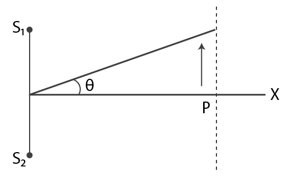Solution:

f = 600 Hz and v = 330 m/s

We know, v = fλ

or λ = v/f = 330/600 = 0.5 mm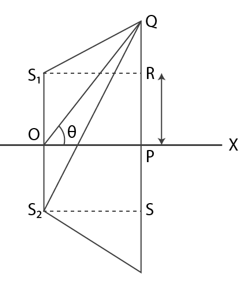Let x be the path difference between the two sound waves reaching the man:

Form figure, x = S2Q – S1 Q = yd/D

Where y = distance travelled by man parallel to y-axis. and d = distance between the two speakers and D = Distance of man from origin.

Also, we are given d = 2m

Now, θ = y/D

(a) For minimum intensity:

x = (2n + 1)(λ/2)

For n = 0

yd/D = λ/2

We know, θ = y/D

=> θd = λ/2

=> θ = λ/2d = 0.55/4 = 0.1375 rad = 7.9o

(b) For maximum intensity:

x = nλ

For n =1

=> yd/D = λ

or θ = λ/d = 0.55/2 = 0.275 rad = 16o

(c) The more number of maxima is given by the path difference:

yd/D = 2λ, 3λ, ….

=> y/D = θ = 32o, 64o , 128o

Therefore, man can hear two more maxima at 32o and 64o because the maximum value of may be at 90o.

Question 32: Three sources of sound S1, S2 and S3 of equal intensity are placed in a straight line with S1S2 = S2S3 (figure below). At a point P, far away from the sources, the wave coming from S2 is 120o ahead in phase of that from S1. Also, the wave coming from S3 is 120o ahead of that from S2. What would be the resultant intensity of sound at P?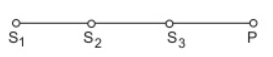Solution:

Since the 3 sources are of the same size, the amplitude is equal to So, A1 = A2 = A3

The resulting amplitude = 0 (By vector method)

So, the resulting intensity at B is zero.

Question 33: Two coherent narrow slits emitting sound of wavelength λ in the same phase are placed parallel to each other at a small separation of 2λ. The sound is detected by moving a detector on the screen ∑ at a distance D(>>λ) from the slit S1 as shown in figure (below). Find the distance x such that the intensity at P is equal to the intensity at O.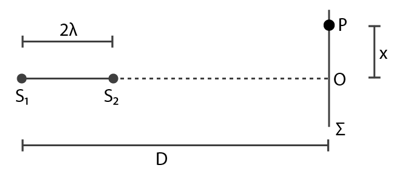Solution:

S1 and S2 are in the same phase. At O, there will be maximum intensity.

There will be maximum intensity at P.

From right angled triangles, ΔS1PO and ΔS2PO

(S1P)2 – (S2P)2

= (D2 + x2) – ((D-2λ)2 + x2) 2

= 4λD + 4λ2

= 4λD

If λ is small, then λ2 is neglisible.

(S1P + S2P)(S1P – S2P) = 4λD

=> (S1P – S2P) = 4λD/[2√(x2+D2)] = nλ

=> 2D/√(x2+D2) = n

or x = (D/n)√(4-n2)

When n = 1, x = √3D

When n = 2, x = 0

When x = √3D, the intensity at P is equal to the intensity at O.

Question 34: Figure (below) shows two coherent sources S1 and S2 which emit sound of wavelength λ in phase. The separation between the sources is 3λ. A circular wire of large radius is placed in such a way that S1S2 lies in its plane and the middle point of S1S2 is at the centre of the wire. Find the angular positions θ on the wire for which constructive takes place.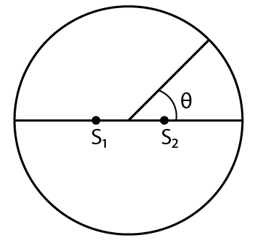Solution:

Let S1 and S2 sound waves from the two coherent sources reach the point P.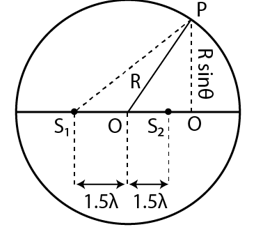From the figure,

PS12 = PQ2 + QS2 = (R sinθ) 2 + (Rcosθ – 1.5 λ)2

PS12 = PQ2 + QS12 = (R sinθ) 2 + (Rcosθ – 1.5 λ)2

Path difference between the sound waves reaching point P:

(S1P)2 – (S2P)2 = [(R sinθ)2 + (Rcosθ + 1.5 λ)2 ] – [(R sinθ)2 + (Rcosθ – 1.5 λ)2 ]

= 6 λ cosθ

=> (S1P – S2P) = 3 λ cosθ = n λ

=> cos θ = n/3

=> θ = cos-1(n/3)

Where n = 0, 1, 2, ….

θ = 0°, 48.2°, 70.5°and 90° are similar points in other quadrants.

Question 35: Two sources of sound S1 and S2 vibrate at same frequency and are in phase (figure below). The intensity of sound detected at a point P as shown in the figure is I0.

(a) If θ equals 45°, what will be the intensity of sound detected at this point if one of the Sources is switched off?

(b) What will be the answer of the previous part if θ = 60o?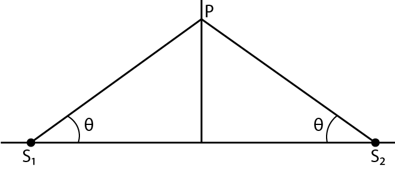Solution:

(a) When θ = 45°:

Path difference = S1P − S2P = 0

So, when the source is switched off, the intensity of sound at P is Io/4.

(b) When θ = 60o, the path difference is also zero. Similarly, it can be proved that the intensity at P is Io/4 When the source is switched off.